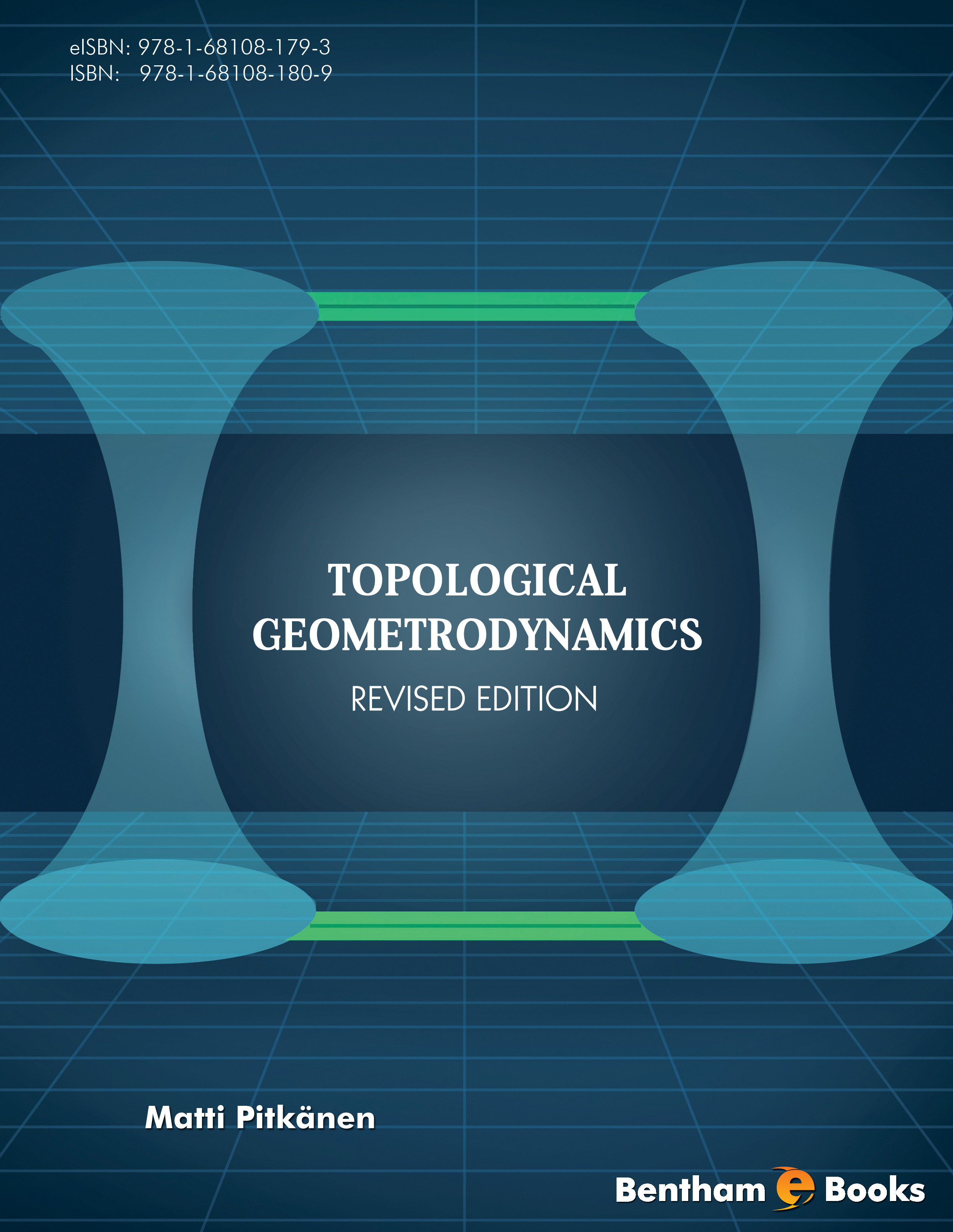### Was Von Neumann Right After All?

#### Abstract

The work with TGD inspired model for quantum computation led to the realization that von Neumann algebras, in particular hyper-finite factors, could provide the mathematics needed to develop a more explicit view about the construction of M-matrix generalizing the notion of S-matrix in zero energy ontology (ZEO). In this chapter I will discuss various aspects of hyper- finite factors and their possible physical interpretation in TGD framework. &lt;/p&gt;&lt;p&gt; 1. Hyper- finite factors in quantum TGD &lt;/p&gt;&lt;p&gt; The following argument suggests that von Neumann algebras known as hyper- finite factors (HFFs) of type III1 appearing in relativistic quantum field theories provide also the proper mathematical framework for quantum TGD. &lt;/p&gt;&lt;p&gt; 1. The Clifford algebra of the infinite-dimensional Hilbert space is a von Neumann algebra known as HFF of type II1. Therefore also the Clifford algebra at a given point (light-like 3-surface) of world of classical worlds (WCW) is HFF of type II1. If the fermionic Fock algebra defined by the fermionic oscillator operators assignable to the induced spinor fields (this is actually not obvious!) is infinite-dimensional it defines a representation for HFF of type II1. Super-conformal symmetry suggests that the extension of the Clifford algebra defining the fermionic part of a super-conformal algebra by adding bosonic super-generators representing symmetries of WCW respects the HFF property. It could however occur that HFF of type II&amp;#8734; results. &lt;/p&gt;&lt;p&gt; 2. WCW is a union of sub-WCWs associated with causal diamonds (CD) defined as intersections of future and past directed light-cones. One can allow also unions of CDs and the proposal is that CDs within CDs are possible. Whether CDs can intersect is not clear......

Total Pages: 677-797 (121)